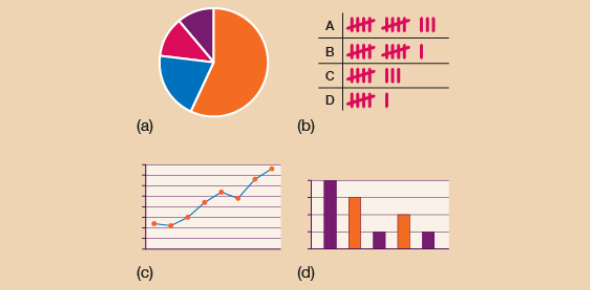# Discrete Vs Continuous Quiz

12 Questions | Attempts: 53309SettingsData can be described in two ways, i. E. , discrete and continuous. Discrete data can take on only integer values, whereas continuous data can take on any value. Are you ready for the Discrete Vs. Continuous quiz? This discrete or continuous quiz below is designed to assess and reinforce the student's understanding of the nature and differences between discrete and continuous data. Give it a try and see how well you understand it!

• 1.
The qualities of discrete data can be
• A.

Measured

• B.

Counted

• C.

Both

• D.

None

• 2.
The qualities of continuous data can be
• A.

Measured

• B.

Counted

• C.

Both

• D.

None

• 3.
Which of these is NOT continuous data:
• A.

A person's weight each week

• B.

The volume of water in the pacific ocean each day

• C.

Bikes manufactured in a factory each day

• D.

None of these

• 4.
Which of these is NOT discrete data:
• A.

Weight of a watermelon  as measured each week

• B.

How many students attend the class

• C.

How many cars a company sells each day.

• D.

None of these

• 5.
Daily rainfall is an example of what sort of data:
• A.

Discrete

• B.

Continuous

• C.

Both

• D.

None

• 6.
The distance that a cyclist rides each day is what sort of data:
• A.

Discrete

• B.

Continuous

• C.

Both

• D.

None

• 7.
The frequency of a cyclist riding over a few kms weekly is this sort of data.
• A.

Discrete

• B.

Continuous

• C.

Both

• D.

None

• 8.
The number of coconuts produced by a coconut tree each year is continuous data.
• A.

True

• B.

False

• 9.
The average size of the coconut grown by a tree is continuous data.
• A.

True

• B.

False

• 10.
The combined tonnage of mail passing each day through the local postal center is discrete data:
• A.

True

• B.

False

• 11.
Which of these is continuous data?
• A.

The dog weighs 46.6 kg.

• B.

The dog has four legs.

• C.

The dog has one tail.

• D.

The dog has two ears.

• 12.
Which of these is discrete data?
• A.

Paul weighs 99.3 kg.

• B.

Paul has three sisters.

• C.

Paul is 167 cm tall.

• D.

Paul jumps 204 cm high.

## Related TopicsBack to top
×

Wait!
Here's an interesting quiz for you.